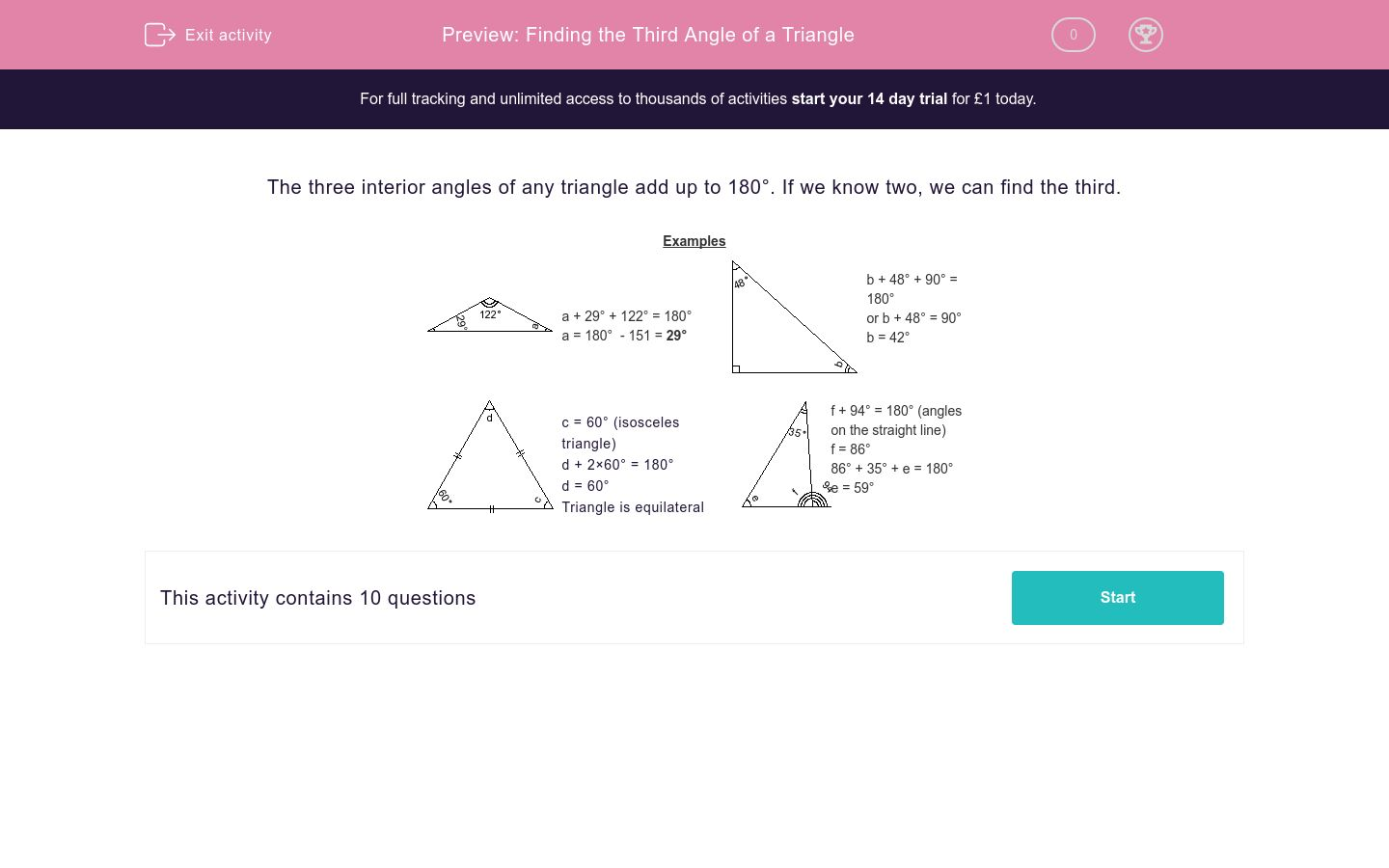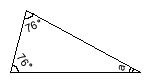# Finding the Third Angle of a Triangle

In this worksheet, students find the third angle of a triangle given the other two angles.Key stage:  KS 2

Curriculum topic:  Maths and Numerical Reasoning

Curriculum subtopic:  2D Shapes: Circles, Angles and Bearings

Difficulty level:### QUESTION 1 of 10

The three interior angles of any triangle add up to 180°. If we know two, we can find the third.

Examplesa + 29° + 122° = 180° a = 180°  - 151 = 29°b + 48° + 90° = 180° or b + 48° = 90° b = 42°c = 60° (isosceles triangle) d + 2×60° = 180° d = 60° Triangle is equilateralf + 94° = 180° (angles on the straight line) f = 86° 86° + 35° + e = 180° e = 59°

Find the missing angle in this triangle.a = 22°

a = 28

a = 28°

Find the missing angle in this triangle.b = 22°

b = 42°

b = 31°

Find the missing angle in this triangle.b = 30°

b = 20°

b = 15°

Find the missing angles in this triangle.c = 90°

c = 60°

d = 60°

d = 90°

Find the missing angle in this triangle.a = 35°

a = 47°

a = 37°

Find the missing angles in this triangle.e = 47°

e = 74°

e = 72°

f = 47°

f = 74°

Find the missing angle in this triangle.a = 45°

a = 90°

a = 53°

Find the missing angle in this triangle.a = 45°

a = 30°

a = 29°

Find the missing angle in this triangle.f = 73°

f = 37°

f = 107°

e = 43°

e = 34°

e = 73°

Find the missing angle in this triangle.c = 41°

c = 14°

e = 89°

e = 98°

• Question 1

Find the missing angle in this triangle.a = 28°
EDDIE SAYS
a + 76 + 76 = 180 180 - 76 - 76
• Question 2

Find the missing angle in this triangle.b = 42°
EDDIE SAYS
b + 90 + 48 = 180
b = 180 - 90 - 48 b = 42
• Question 3

Find the missing angle in this triangle.b = 30°
EDDIE SAYS
b + 90 + 60 = 180
b = 180 - 90 - 60 b = 30
• Question 4

Find the missing angles in this triangle.c = 60°
d = 60°
EDDIE SAYS
All three sides are equal so all the angles must be the same
• Question 5

Find the missing angle in this triangle.a = 37°
EDDIE SAYS
a + 90 + 53 = 180
a = 180 - 90 - 53 a = 37
• Question 6

Find the missing angles in this triangle.e = 74°
f = 74°
EDDIE SAYS
Angles on a straight line must add to 180 ° f + 106 = 180
f = 74
Angles in a triangle add to 180 e + 74 + 32 = 180 e = 180 - 74 - 32 e = 74
• Question 7

Find the missing angle in this triangle.a = 53°
EDDIE SAYS
a + 90 + 37 = 180
a = 180 - 90 - 37 a = 53
• Question 8

Find the missing angle in this triangle.a = 29°
EDDIE SAYS
a + 122 + 29 = 180
a = 180 - 122 - 29 a = 29
• Question 9

Find the missing angle in this triangle.f = 73°
e = 34°
EDDIE SAYS
Angles on a straight line add up to 180 f = 180 - 107 = 73
Angles in a triangle add to 180 e = 180 - 73 - 73 e = 34
• Question 10

Find the missing angle in this triangle.c = 41°
e = 98°
EDDIE SAYS
Two sides are equal (the little dashes on the side) so two angles are equal. c = 41
e = 180 - 41 - 41 e = 98
---- OR ----

Sign up for a £1 trial so you can track and measure your child's progress on this activity.

### What is EdPlace?

We're your National Curriculum aligned online education content provider helping each child succeed in English, maths and science from year 1 to GCSE. With an EdPlace account you’ll be able to track and measure progress, helping each child achieve their best. We build confidence and attainment by personalising each child’s learning at a level that suits them.

Get started#### Similar activities you may like

Start your £1 trial today.
Subscribe from £10/month.